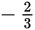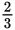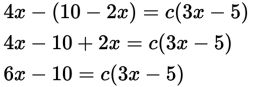# SAT Math Multiple Choice Question 250: Answer and Explanation

### Test Information

Question: 250

10. 4x - (10 - 2x) = c (3x - 5)

If the equation shown has infinitely many solutions, and c is a constant, what is the value of c?

• A. -2
• B.• C.• D. 2

Explanation:

D

Difficulty: Medium

Category: Heart of Algebra / Linear Equations

Strategic Advice: There are two variables and only one equation, so you can't actually solve the equation for c. Instead, recall that an equation has infinitely many solutions when the left side is identical to the right side. When this happens, everything cancels out and you get the equation 0 = 0, which is always true.

Getting to the Answer: Start by simplifying the left side of the equation. Don't forget to distribute the negative sign to both terms inside the parentheses.Next, quickly compare the left side of the equation to the right side. Rather than distributing the c, notice that if c were 2, then both sides of the equation would equal 6x - 10, and it would have infinitely many solutions. Therefore, c is 2.